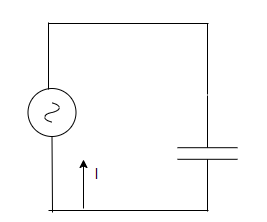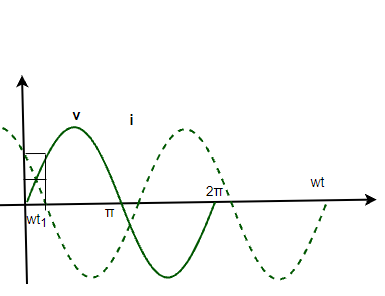# AC Voltage Applied to a Capacitor

• Last Updated : 20 Oct, 2021

Alternating Currents and Voltages vary and change their directions with time. They are widely used in modern-day devices and electrical systems because of their numerous advantages. Circuits in everyday life consist of resistances, capacitors, and inductances. Capacitors are the devices that accumulate charges on their plates and store the charges. It is essential to understand the behavior of the circuit when capacitance is connected to a voltage source.

### AC Voltage Applied to a Capacitor

The figure given below shows an AC circuit. Here, an AC voltage source is connected to a capacitor. The expression for the voltage from the voltage source is given by v = vmsin(ωt). A capacitor is an electrical device that stores electrical energy. It is a passive electronic component with two terminals. The effect of the capacitor is known as capacitance. A capacitor when connected to a voltage source draws current from the source so as to charge itself. Once the capacitor is charged, the potential at its plates becomes equal to the potential at the battery. At this point, the current stops flowing into the capacitor. This is called the charging of the capacitor.

Attention reader! All those who say programming isn't for kids, just haven't met the right mentors yet. Join the  Demo Class for First Step to Coding Course, specifically designed for students of class 8 to 12.

The students will get to learn more about the world of programming in these free classes which will definitely help them in making a wise career choice in the future.

In case a charged capacitor is put in a circuit where the potential at the plates of the capacitors is greater than the potential at the voltage source. In that case, the capacitor starts acting like a voltage source with varying voltage. The current starts flowing from the capacitor and thus decreasing the charge on its plates. This is called discharging of the capacitor.In the circuit given above, the current will flow for a short time during which the capacitor charges. As it charges, the current decreases. In situations where a capacitor is connected to an ac source, it regulates the current but does not completely prevent the flow of charge. The capacitor is alternately discharged and charged as the direction of the current is reversed at every half cycle.

At a particular time “t”, denoting the charge on the capacitor by “q”. The instantaneous voltage across the capacitor is given by,Using the kirchoff’s rule,Since the current is continuously changing, to find the the current. Derivative of the charge is required,

Differentitating the given equation,⇒⇒i = vmωC cos(ωt)

Rearranging the above equation,

i = imsin(ωt + π/2)

Here, im = vmωC. It is the amplitude of the oscillating current. It can also be re-written as,This equation when compared to the ohm’s law gives 1/ωC as resistance. It is called capacitive reactance and it is denoted by XC

Now, the amplitude of the current becomes,

imThe dimensions of capacitive reactance are the same as resistance and its SI unit is Ohms. Intuitively speaking, capacitive reactance limits the current of a purely capacitive circuit in the same way as resistance limits the current in a usual resistive circuit.

Previous equations show that current is ahead of voltage in terms of phase. There is a phase difference of π/2. The figure given below shows the variation of voltage and current with time.The power dissipated in a purely capacitive circuit can be derived using the instantaneous equation of power,

Pc = iv

⇒ Pc = (imsin(ωt + π/2))(vmsin(ωt))

⇒ Pc = imvmcos(ωt)sin(ωt)

⇒ Pc =  imvm/2sin(2ωt)

The average power dissipated in this case,

Pav = 0

### Sample Problems

Question 1: A capacitor of 12pF is connected to a voltage source of frequency 50Hz. Find the reactance of the capacitance.

The reactance of the capacitance is given by,

X = 1/ωC

Given:

f = 50Hz

C = 12 pF

ω = 2πf

⇒ ω = 2π(50)

⇒ ω = 100π

Plugging the values in the equation,

X = 1/ωC

⇒ X = 1/(100π × 12 × 10-12)

⇒ X = 1/(12π × 10-10)

⇒ X = 0.0265 × 1010

⇒ X = 2.65 × 108 Ohms

Question 2: A capacitor of 24pF is connected to a voltage source of frequency 50Hz. Find the reactance of the capacitance.

The reactance of the capacitance is given by,

X = 1/ωC

Given:

f = 50Hz

C = 24 pF

ω = 2πf

⇒ ω = 2π(50)

⇒ ω = 100π

Plugging the values in the equation,

X = 1/ωC

⇒ X = 1/(100π × 24 × 10-12)

⇒ X = 1/(24π × 10-10)

⇒ X = 0.01325 × 1010

⇒X = 1.325 × 108 Ohm

Question 3: A capacitor of 1000 pF is connected to a voltage source given by,

v = 50sin(20t)

Find the amplitude of the current.

The reactance of the capacitance is given by,

XC = 1/ωC

Given:

ω = 20

C = 10pF

Plugging the values in the equation,

XC = 1/ωC

⇒ XC = 1/(20 × 1000 ×10-12)

⇒ XC = 1/(2 × 10-8)

⇒ XC = 0.5 × 108

⇒ XC = 5 × 107 Ohm

Amplitude of the current will be,

im = vm/Xc

⇒ im = 50 / (5 ×  107)

⇒ im = 10-6A

Question 4: A sinusoidally varying current is applied to a capacitive circuit. The impedance of the capacitance is given as 2 ohms. Find the power dissipated in the circuit if the voltage source has an RMS voltage of 45V.

The Average power is given by,

P = VIcos(φ)

Since the circuit is purely capcitive circuit. The phase angle will be 90°.

cos(φ) = 0

Plugging the values in the equation,

P = VIcos(φ)

P = 0.

Question 5: A capacitor of 10pF is connected to a voltage source given by,

v = 50sin(20t)

Find the amplitude of the current.

The reactance of the capacitance is given by,

XC = 1/ωC

Given:

ω = 20

C = 10pF

Plugging the values in the equation,

XC = 1/ωC

⇒ XC = 1/(20 × 10 ×10-12)

⇒ XC = 1/(2 × 10-10)

⇒ XC = 0.5 × 1010

⇒ XC = 5 × 109 Ohm

Amplitude of the current will be,

im = vm/Xc

⇒ im = 50 / (5 ×  109)

⇒ im = 10-8A

My Personal Notes arrow_drop_up
Recommended Articles
Page :